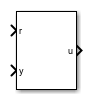# Active Disturbance Rejection Control

Design controller for plants with unknown dynamics and disturbances

• Library:

•## Description

The Active Disturbance Rejection Control block lets you design active disturbance rejection control (ADRC) for a plant with unknown dynamics and internal and external disturbances. ADRC is a model-free control technique that requires only an approximation of the plant dynamics to design controllers that provide robust disturbance rejection.

The block uses a first-order or second-order model approximation of the known system dynamics along with the unknown dynamics and disturbances modeled as an extended state of the plant. Typically, you determine this order from the open-loop step response of your plant in the operating range.

• First-order approximation — $\stackrel{˙}{y}\left(t\right)={b}_{0}u\left(t\right)+f\left(t\right)$

• Second-order approximation — $\stackrel{¨}{y}\left(t\right)={b}_{0}u\left(t\right)+f\left(t\right)$

Here:

• y(t) is the plant output.

• u(t) is the input signal.

• b0 is the critical gain, which is the estimated gain that describes the plant response to an input u(t).

• f(t) is the total disturbance, which includes unknown dynamics and other disturbances.

The block uses an extended state observer (ESO) to estimate f(t) and implements disturbance rejection control by reducing the effect of estimated disturbances on the known part of model approximation. To tune ADRC, set appropriate time domain, model type and critical gain, controller and observer bandwidths, and initial conditions.

## Ports

### Input

expand all

Provide the reference signal for the controlled system to follow.

Provide the output signal of the plant.

### Output

expand all

Connect the control input signal to the plant input.

Estimated extended states of the plant model from the extended state observer.

If the Model type is , xhat is a vector of length two, with estimated states $\stackrel{^}{y}$ and $\stackrel{^}{f}\left(t\right)$.

If the Model type is , xhat is a vector of length three, with estimated states $\stackrel{^}{y}$, $\stackrel{^}{\stackrel{˙}{y}}$, and $\stackrel{^}{f}\left(t\right)$.

#### Dependencies

To enable this output port, select the Estimated extended states parameter.

## Parameters

expand all

### Parameters Tab

Specify the controller time domain.

When you select discrete-time, specify the sample time using the Sample time parameter.

#### Programmatic Use

 Block Parameter: `'time_domain'` Type: character vector Values: `'discrete-time'` | `'continuous-time'` Default: `'discrete-time'`

Specify the sample time value for the discrete-time controller.

#### Dependencies

To enable this parameter, set the Time domain parameter to discrete-time.

#### Programmatic Use

 Block Parameter: `'Ts'` Type: character vector Values: finite positive scalar Default: `'0.01'`

Specify the model type of your plant as one of the following.

• first-order — Select this option if your plant exhibits first-order dynamic system behavior.

• second-order — Select this option if your plant exhibits second-order dynamic system behavior.

#### Programmatic Use

 Block Parameter: `'model_type'` Type: character vector Values: `'first-order'` | `'second-order'` Default: `'first-order'`

Specify the critical gain b0 that describes the model behavior.

#### Programmatic Use

 Block Parameter: `'b0'` Type: character vector Values: finite nonzero scalar Default: `'1'`

Specify the controller bandwidth. This parameter determines the speed of the controller response. In general, a faster response requires a larger controller bandwidth.

#### Programmatic Use

 Block Parameter: `'wc'` Type: character vector Values: finite positive scalar Default: `'1'`

Specify the observer bandwidth. Typically, this is set to 5 to 10 times the controller bandwidth so that the observer converges faster than the controller.

#### Programmatic Use

 Block Parameter: `'wo'` Type: character vector Values: finite positive scalar Default: `'10'`

### Block Tab

Specify the initial state values for extended state observer as a scalar or vector of length n.

If Model type is , n = 2. Otherwise, n = 3.

#### Programmatic Use

 Block Parameter: `'x0'` Type: character vector Values: finite scalar | vector Default: `'0'`

Option to limit block output to specified saturation limits. Specify the output saturation limits using the Upper limit and Lower limit parameters.

#### Programmatic Use

 Block Parameter: `'ulim_checkbox'` Type: character vector Values: `'off'` | `'on'` Default: `'off'`

Specify the upper limit for the block output. The block output is held at this value whenever it would otherwise exceed this value.

#### Dependencies

To enable this parameter, select the Limit output parameter.

#### Programmatic Use

 Block Parameter: `'umax'` Type: character vector Values: scalar Default: `'inf'`

Specify the lower limit for the block output. The block output is held at this value whenever it would otherwise go below this value.

#### Dependencies

To enable this parameter, select the Limit output parameter.

#### Programmatic Use

 Block Parameter: `'umin'` Type: character vector Values: scalar Default: `'–inf'`

Option to output states from the extended state observer.

If the Model type is , the block outputs $\stackrel{^}{y}$ and $\stackrel{^}{f}\left(t\right)$.

If the Model type is , the block outputs $\stackrel{^}{y}$, $\stackrel{^}{\stackrel{˙}{y}}$, and $\stackrel{^}{f}\left(t\right)$.

#### Programmatic Use

 Block Parameter: `'xhat_checkbox'` Type: character vector Values: `'off'` | `'on'` Default: `'off'`

## Version History

Introduced in R2022b